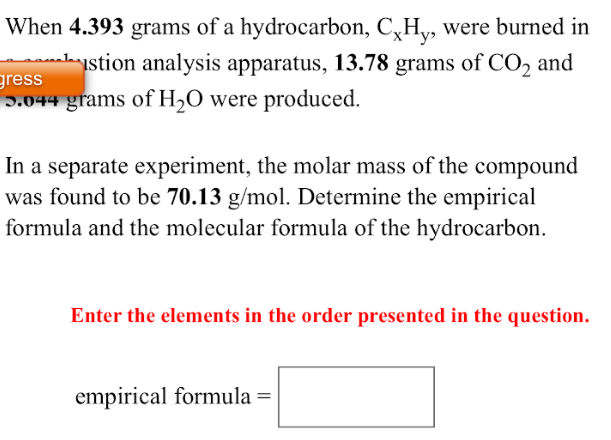# When 4.393 grams of a hydrocarbon, CxHy, were burned in a combustion analysis apparatus, 13.78 grams of CO2 and 5.044 grams of H2O were produced. In a separate experiment, the molar mass of the compound was found to be 70.13 g/mol. Determine the empirical formula and the molecular formula and the molecular formula of the hydrocarbon. Enter the elements in the order presented in the question. Empirical formula=